# Dunford integral

(diff) ← Older revision | Latest revision (diff) | Newer revision → (diff)

An integral playing a key role in the Riesz–Dunford functional calculus for Banach spaces (cf. Functional calculus.) In this calculus, for a fixed bounded linear operatoron a Banach space, all functionsholomorphic on a neighbourhoodof the spectrum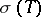of(cf. also Spectrum of an operator) are turned into a bounded linear operatoronbyThis integral is called the Dunford integral. It is assumed here that the boundary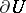ofconsists of a finite number of rectifiable Jordan curves (cf. also Jordan curve), oriented in positive sense.

For suitably chosen domains ofand, the following rules of operational calculus hold: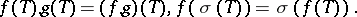Also,onimplies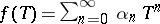in the operator norm. If, then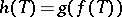.

The Dunford integral can be considered as a Bochner integral.

How to Cite This Entry:
Dunford integral. Encyclopedia of Mathematics. URL: http://encyclopediaofmath.org/index.php?title=Dunford_integral&oldid=12378
This article was adapted from an original article by J. de Graaf (originator), which appeared in Encyclopedia of Mathematics - ISBN 1402006098. See original article